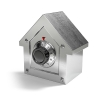#### You may also like### Always Two

Find all the triples of numbers a, b, c such that each one of them plus the product of the other two is always 2.### Symmetricality

Five equations and five unknowns. Is there an easy way to find the unknown values?### A Change in Code

Can you find the percentage increase if the results appear in code?

# Is There More to Discover about Four Consecutive Numbers?

##### Age 14 to 16Challenge Level

This problem follows on from, Continuing to Explore Four Consecutive Numbers.

Take four consecutive numbers, $a$, $b$, $c$, $d$.

1. What is $(a^2 + d^2) - (b^2 + c^2)$ equal to?  Show that it always takes this value.

2. Why is $(a^2 + b^2 + c^2 + d^2) - (1+2+3)$ always divisible by $4$?
Why is it always divisible by $8$?

3. Why is $abcd$ divisible by $24$?

4. Explore $\sqrt{abcd + 1}$.

With thanks to Don Steward, whose ideas formed the basis of this problem.